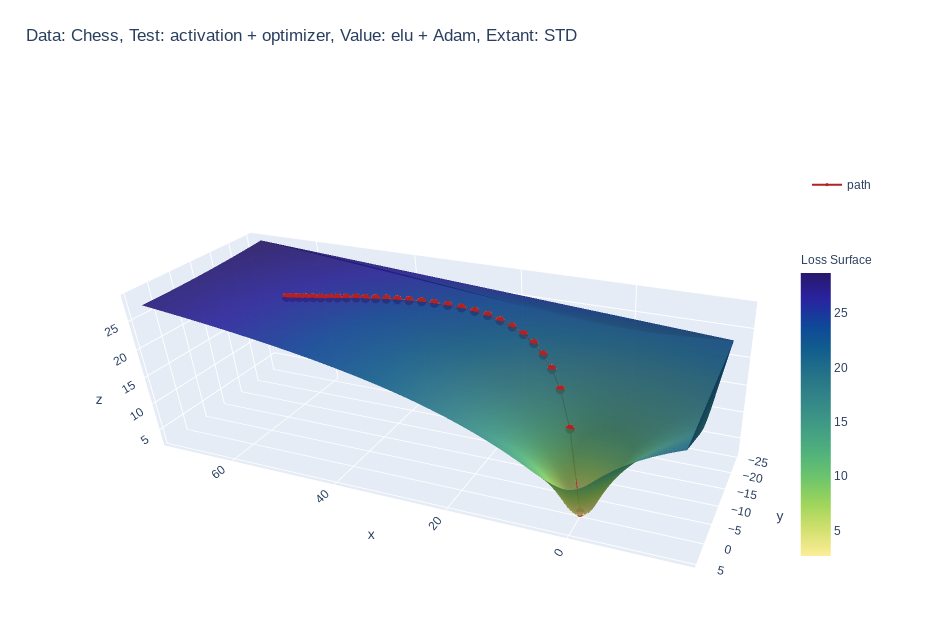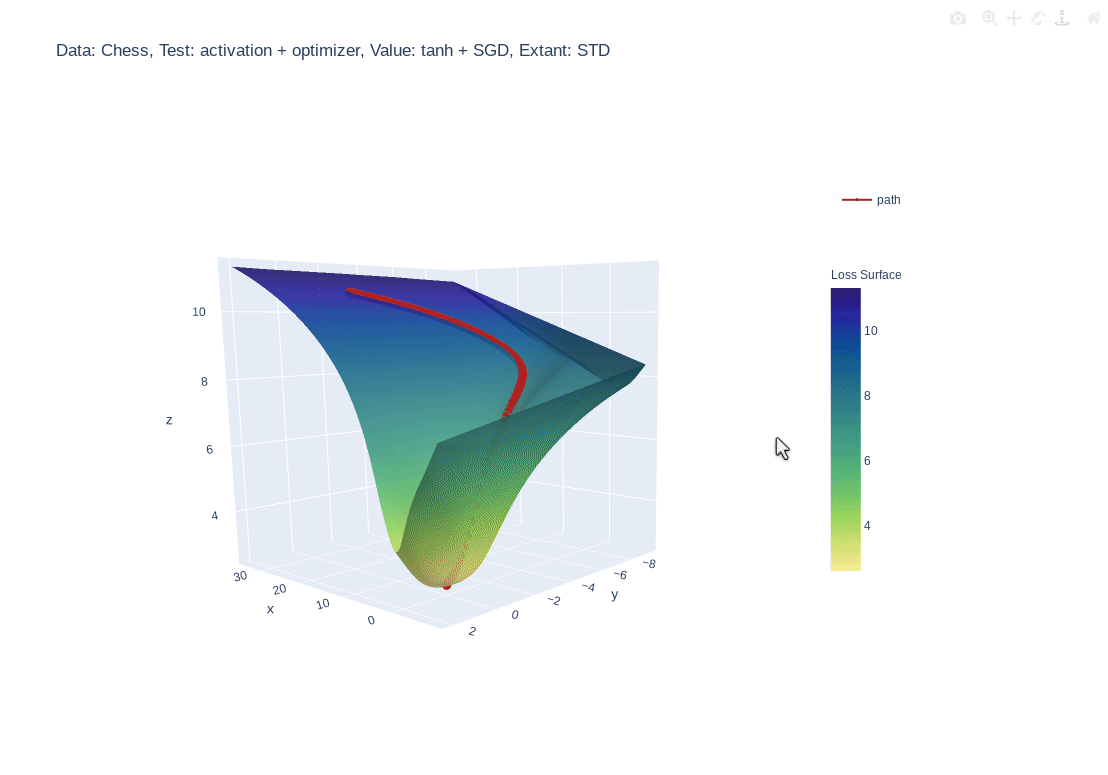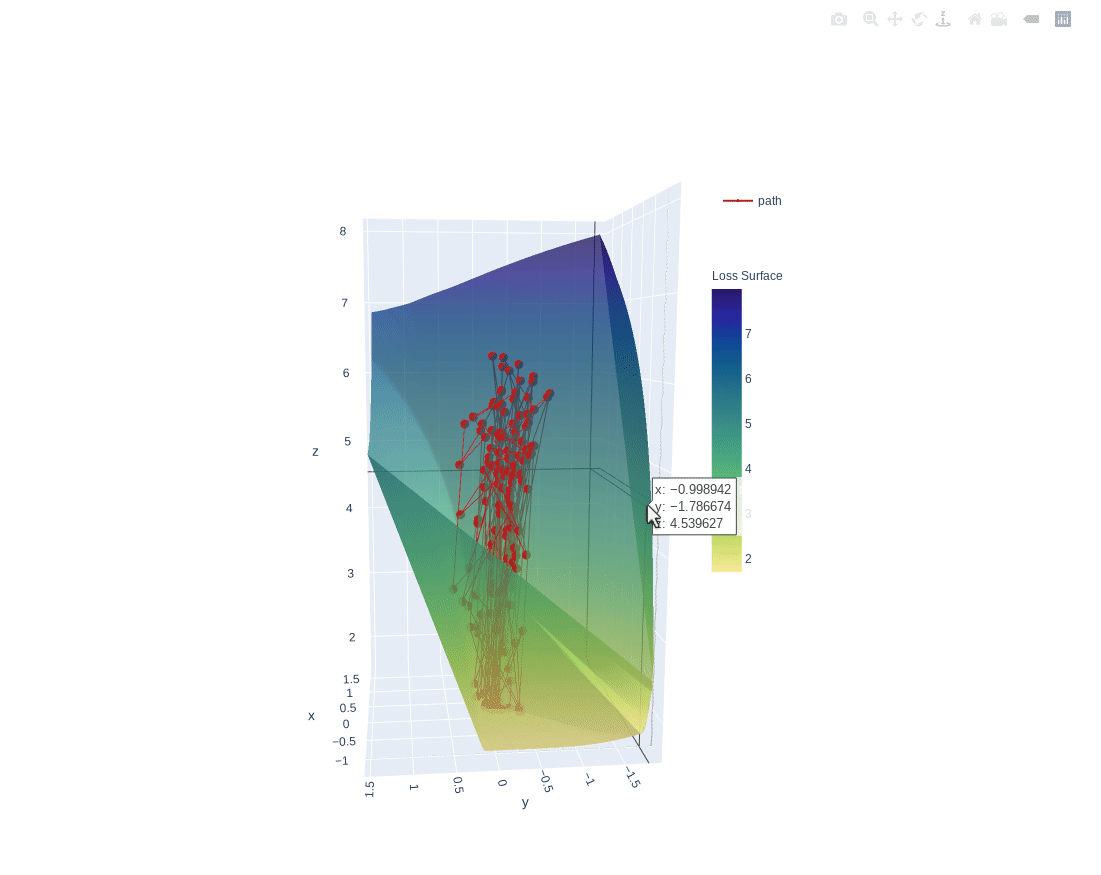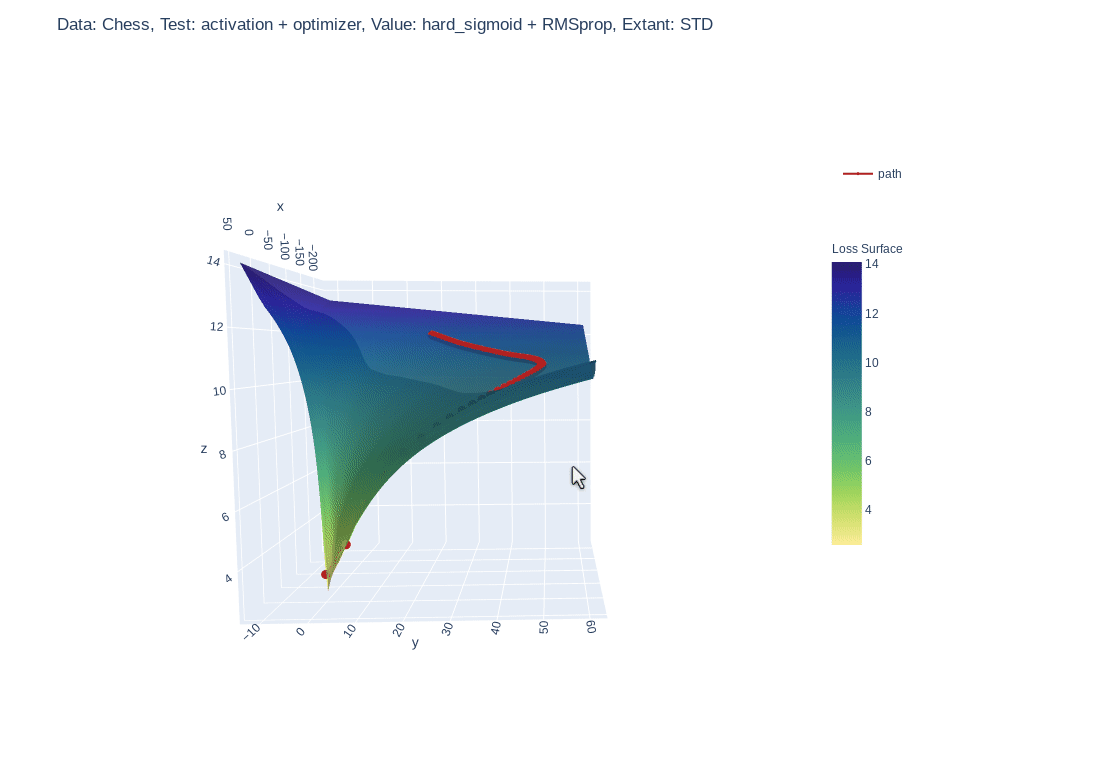## MLVTK

A loss surface visualization tool.Simple feed-forward network trained on chess data, using elu activation and Adam optimizerSimple feed-forward network trained on chess data, using tanh activation and SGD optimizer3 layer feed-forward network trained on hand written letters data, using relu activation, SGD optimizer and learning rate of 2.0. Example of what happens to path when learning rate is too highSimple feed-forward network trained on chess data, using hard-sigmoid activation and RMSprop optimizer

## Why?

• :shipit: Simple: A single line addition is all that is needed.

• :question: Informative: Gain insight into what your model is seeing.

• :notebook: Educational: See how your hyper parameters and architecture impact your

models perception.

## Quick Start

Requiresversion
python>= 3.6.1
tensorflow>= 2.3.1
plotly>=4.9.0

Install locally (Also works in google Colab!):

``````pip install mlvtk
``````

Optionally for use with jupyter notebook/lab:

## Notebook

``````pip install &quot;notebook&gt;=5.3&quot; &quot;ipywidgets==7.5&quot;
``````

## Lab

``````pip install jupyterlab &quot;ipywidgets==7.5&quot;

# Basic JupyterLab renderer support
jupyter labextension install [email&#160;protected]

# OPTIONAL: Jupyter widgets extension for FigureWidget support
jupyter labextension install @jupyter-widgets/jupyterlab-manager [email&#160;protected]
``````

### Basic Example

``````from mlvtk.base import Vmodel
import tensorflow as tf
import numpy as np

# NN with 1 hidden layer
inputs = tf.keras.layers.Input(shape=(None,100))
dense_1 = tf.keras.layers.Dense(50, activation='relu')(inputs)
outputs = tf.keras.layers.Dense(10, activation='softmax')(dense_1)
_model = tf.keras.Model(inputs, outputs)

# Wrap with Vmodel
model = Vmodel(_model)
model.compile(optimizer=tf.keras.optimizers.SGD(),
loss=tf.keras.losses.CategoricalCrossentropy(), metrics=['accuracy'])

# All tf.keras.(Model/Sequential/Functional) methods/properties are accessible
# from Vmodel

model.summary()
model.get_config()
model.get_weights()
model.layers

# Create random example data
x = np.random.rand(3, 10, 100)
y = np.random.randint(9, size=(3, 10, 10))
xval = np.random.rand(1, 10, 100)
yval = np.random.randint(9, size=(1,10,10))

# Only difference, model.fit requires validation_data (tf.data.Dataset, or
# other container
history = model.fit(x, y, validation_data=(xval, yval), epochs=10, verbose=0)

# Calling model.surface_plot() returns a plotly.graph_objs.Figure
# model.surface_plot() will attempt to display the figure inline

fig = model.surface_plot()

# fig can save an interactive plot to an html file,
fig.write_html(&quot;surface_plot.html&quot;)

# or display the plot in jupyter notebook/lab or other compatible tool.
fig.show()
``````

## GitHub

https://github.com/tm-schwartz/mlvtk